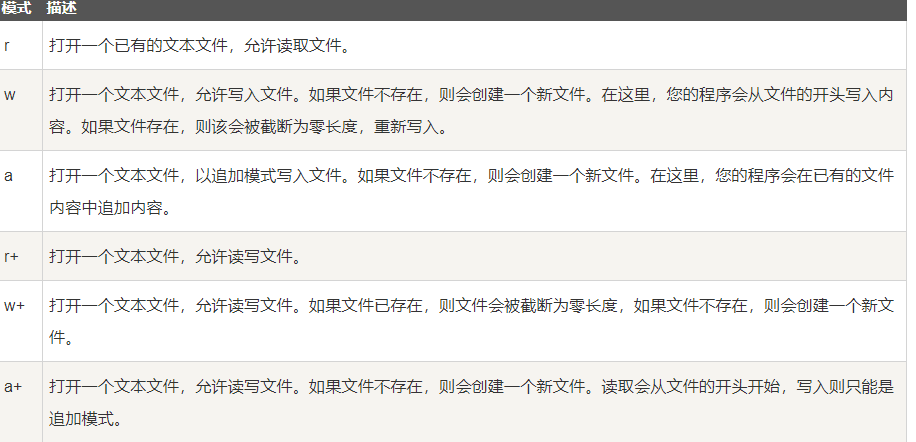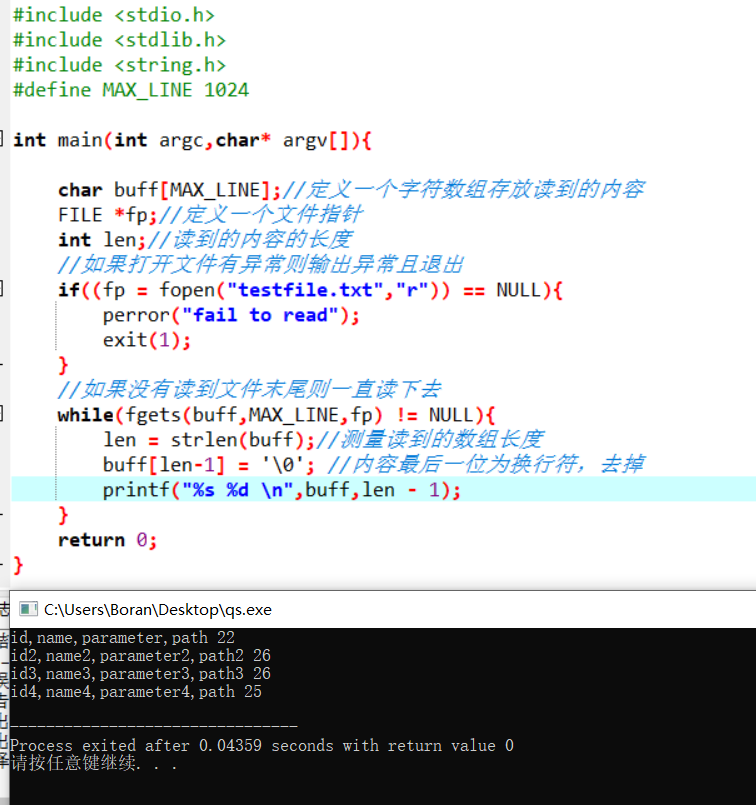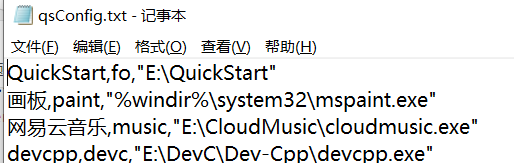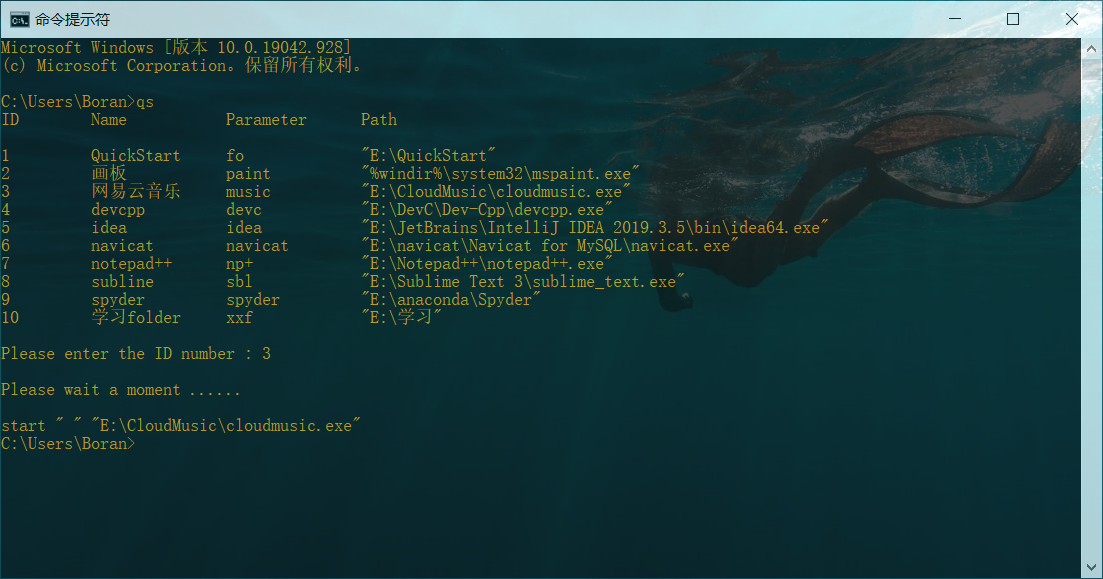4 篇文章 0 订阅

# 过程

## 需求分析

/*
* 需求分析
*	在一个文件中添加文件名与路径还有自定义启动参数
*	此程序带参数 s 启动后会输出一个列表：
*		-------------QuickStart-------------
*		ID	name	parameter	path
*		1   test    test     E/test/test.exe
*		2   test1    test1     E/test/test.exe1
*		3   test2    test2     E/test/test.exe2
*		..   ..    .....     ..............
*	输入序号即可快速启动
*	若带别的参数如test ，则直接启动test
*/


## 学习

### c语言命令行参数的了解学习

c语言main函数有两个参数 int argc,char *argc[]，第一个参数为命令行参数的个数+1（因为包含程序本身），不需要用户传递，第二个参数为一个字符串数组,存储传进来的参数，argv为程序名。

		void main(int argc,char** argv)
{
printf("%d\n",argc);
printf("%s\n",argv);
printf("%s\n",argv);
printf("%s\n",argv);
}


### c语言读取文件的学习

   	FILE *fp = NULL;
fp = fopen("testfile.txt", "r");char buff;
FILE *fp = NULL;
fp = fopen("testfile.txt", "r");
gets(buff,255,fp);### 字符串切割的学习

https://blog.csdn.net/lell3538/article/details/48011135

#include <stdio.h>
#include <stdlib.h>
#include <string.h>
#define MAX_LINE 1024

typedef struct{
char **str;
size_t num;
}IString;

int Split(char *src, char *delim, IString* istr)
{
//src 要拆分的字符串
//delim 分隔符
//istr 返回拆分后的字符串数组与字符串的个数

int i;
char *str = NULL, *p = NULL;

(*istr).num = 1;
str = (char*)calloc(strlen(src)+1,sizeof(char));
if(str == NULL){
return 0;
}
(*istr).str = (char**)calloc(1,sizeof(char *));
if((*istr).str == NULL){
return 0;
}
strcpy(str,src);

p = strtok(str,delim);
(*istr).str = (char*)calloc(strlen(p)+1,sizeof(char));
if((*istr).str == NULL){
return 0;
}
strcpy((*istr).str,p);
for(i = 1; p = strtok(NULL,delim); i++){
(*istr).num ++;
(*istr).str = (char**)realloc(
(*istr).str,
(i+1)*sizeof(char*)
);//首先扩充了字符串数组
if((*istr).str == NULL){
return 0;
}
(*istr).str[i] = (char*)calloc(strlen(p)+1,sizeof(char));
if((*istr).str[i] == NULL){//这个地方将作者的0改为了i
return 0;
}
strcpy((*istr).str[i],p);
}
free(str);
str = p = NULL;
return 1;

}



• strlen(NULL)
得不出结果，且结果不能参与运算
• calloc(num,size)
在内存的动态存储区中分配num个长度为size的连续空间，函数返回一个指向分配起始地址的指针，如果分配不成功，返回NULL
• size_t
long long unsinged int型
• strtok(s, delim)
从s开头开始的一个个被分割的串。当s中的字符查找到末尾时，返回NULL。
strtok(）用来将字符串分割成一个个片段。参数s指向欲分割的字符串，参数delim则为分割字符串中包含的所有字符。当strtok()在参数s的字符串中发现参数delim中包含的分割字符时,则会将该字符改为’\0’字符。在第一次调用时，strtok()必需给予参数s字符串，往后的调用则将参数s设置成NULL。每次调用成功则返回指向被分割出片段的指针。
• realloc(要改变内存大小的指针名，新的大小)，
• NULL在C++中指向0

### c语言中执行dos命令

system("dos 命令");


### 字符串的连接

char pathf[MAX_LINE];
strcpy(pathf,(char*)"\nstart \" \" ");//使用strcpy方法为pathf初始化
//printf(pathf);//测试
strcat(pathf,path);
printf(pathf);
system(pathf);
//其中MAX_LINE为设置的常量


## 整合

### 大概设计

/*
*	定义一个三维的字符数组***char，
*	这个三维数组的长度应是动态可变的
*	将文件按行读入，每读入一行便进行字符串的切割，
*	切割符号为",";
*	将切割完成的字符串数组保存在三维数组中，
*	动态增加三维数组的长度
*	调用时输入的数字为三维数组的下标
*/


### 整合代码

#include <stdio.h>
#include <stdlib.h>
#include <string.h>
#define MAX_LINE 1024
#define QSF "E://QuickStart//qsConfig.txt"

typedef struct{
char **str;
size_t num;
}IString;

typedef struct{
char ***reslist;
int size;
}ResList;

ResList r;

int Split(char *src, char *delim, IString* istr)
{
//	printf("Split invoking.........\n");
//src 要拆分的字符串
//delim 分隔符
//istr 返回拆分后的字符串数组与字符串的个数

int i;
char *str = NULL, *p = NULL;

(*istr).num = 1;
str = (char*)calloc(strlen(src)+1,sizeof(char));
if(str == NULL){
return 0;
}
(*istr).str = (char**)calloc(1,sizeof(char *));
if((*istr).str == NULL){
return 0;
}
strcpy(str,src);

p = strtok(str,delim);
(*istr).str = (char*)calloc(strlen(p)+1,sizeof(char));
if((*istr).str == NULL){
return 0;
}
strcpy((*istr).str,p);
for(i = 1; p = strtok(NULL,delim); i++){
(*istr).num ++;
(*istr).str = (char**)realloc(
(*istr).str,
(i+1)*sizeof(char*)
);
if((*istr).str == NULL){
return 0;
}
(*istr).str[i] = (char*)calloc(strlen(p)+1,sizeof(char));
if((*istr).str[i] == NULL){//这个地方将作者的0改为了i
return 0;
}
strcpy((*istr).str[i],p);
}
str = p = NULL;
return 1;

}

int init(ResList* r){

//	printf("init invoking.........\n");
(*r).size = 0;

IString splres;//修改1 将 IString* splres;改为直接声明
char buff[MAX_LINE];
FILE* fp = NULL;
int len,i,j,k;

if(NULL == (fp = fopen(QSF,"r"))){
exit(1);
}

for(i = 1; fgets(buff,MAX_LINE,fp) != NULL; i++){
//	puts(buff);
len = strlen(buff);
if(buff[len-1] == '\n'){//考虑到可能文件的最后一行并没有换行，所以需要做一个判断
buff[len-1] = '\0';//去掉换行
}

Split(buff,(char*)",",&splres);//修改2 传入的时候取地址

(*r).reslist = (char***)realloc((*r).reslist,(i+1)*sizeof(char**));//增加一个抽屉

(*r).reslist[i] = (char**)calloc(3,sizeof(char*)); //每个抽屉申请三个格子

for(j = 0; j < 3; j ++){//给每个格子赋值
//			printf((*splres).str[j]);
//			printf("\n");
(*r).reslist[i][j] = splres.str[j];//修改3 直接取属性
//			printf((*r).reslist[i][j]);
//			printf("\n") ;
}
(*r).size ++;
}

return 0;
}

int executeQs(char* path){
char pathf[MAX_LINE];
strcpy(pathf,(char*)"\nstart \" \" ");
//printf(pathf);
strcat(pathf,path);
printf(pathf);
system(pathf);

return 0;
}

//参数为空的情况下
//输入id，
//通过id获得path，然后执行
int parameterIsNull(){

char* path;

printf("%-10s%-15s%-15s%-50s\n\n","ID","Name","Parameter","Path");

for(int i = 1; i < r.size+1; i ++){

printf("%-10d%-15s%-15s%-50s",i,r.reslist[i],r.reslist[i],r.reslist[i]);
printf("\n");
}

int id;
printf("%s","\nPlease enter the ID number : ");
scanf("%d",&id);

if(id < 1 || id > r.size){
perror("\nId number is not exist");
return 0;
}
printf("%s","\nPlease wait a moment ...... \n");

path = r.reslist[id];

executeQs(path);

return 1;
}

//参数不为空的情况下
//传入参数，通过参数查找id，
//通过id获得path，然后执行
int parameterIsNotNull(char* parameter){
char* path = (char*)"IsNotPath";

for(int i = 1; i < r.size+1; i++){

if(!strcmp(r.reslist[i],parameter)){
path = r.reslist[i];
break;
}

}
if(!strcmp("IsNotPath",path)){
perror("Parameter is not exist");
return 0;
}
printf("%s","\nPlease wait a moment ...... \n");
executeQs(path);

return 1;
}

int main(int argc,char* argv[]){

init(&r);

if(argc == 2){

char* parameter = argv;
parameterIsNotNull(parameter);

}else if(argc == 1){
parameterIsNull();
}else{
perror("Parameter is Error");
}

return 0;
}



### 配置文件示例## 放上最终效果# 一些问题

• 刚开始打算使用计算的方式在最后得到三维数组的长度，也就是得到配置文件中的行数，但一直行不通，计算结果有问题，于是后来改用结构体来记录：读入文件循环的时候动态增加。
• 在代码中定义字符串时会将’‘视为转义字符，如果是从文件中读取则不会将’'视为转义字符。
• 字符串转换为字符指针 (char*)“testString”，直接将字符串赋值给字符指针会有警告。
• 指针是第一次接触学习，所以犯了挺多错误。
• 没有优化内存回收。

02-14
05-2018605-071万+
10-227446
08-251420
04-255835
03-304527
12-03352
06-102323
03-16947
11-132831
11-262254
05-311288
08-192080
08-286528
10-182177
09-113170
12-127589
05-184926点击重新获取扫码支付余额充值